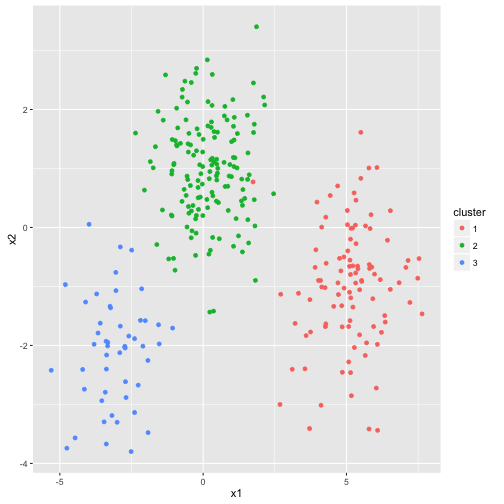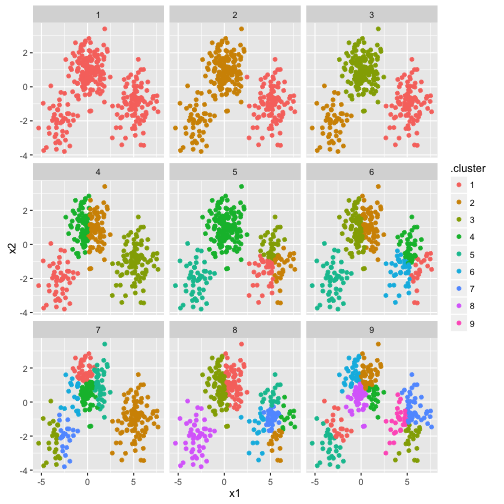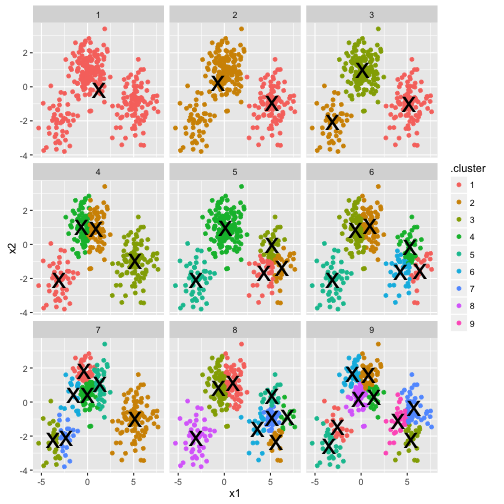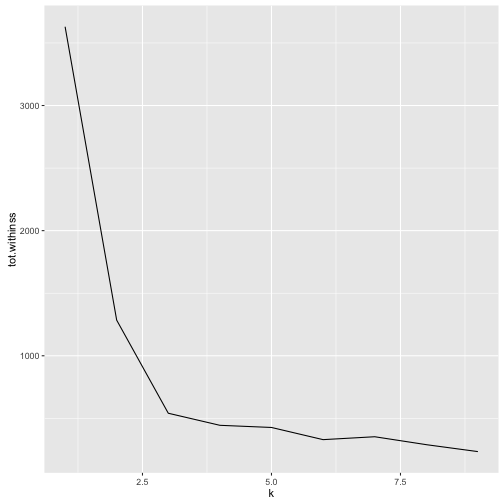# Tidying k-means clustering

K-means clustering serves as a very useful example of tidy data, and especially the distinction between the three tidying functions: `tidy`, `augment`, and `glance`.

Let's start by generating some random 2d data with three clusters, within which points are distributed according to a multivariate gaussian:

``````library(dplyr)

set.seed(2014)
centers <- data.frame(cluster=factor(1:3), size=c(100, 150, 50), x1=c(5, 0, -3), x2=c(-1, 1, -2))
points <- centers %>% group_by(cluster) %>%
do(data.frame(x1=rnorm(.\$size, .\$x1),
x2=rnorm(.\$size, .\$x2)))

library(ggplot2)
ggplot(points, aes(x1, x2, color=cluster)) + geom_point()
``````This is an ideal case for k-means clustering. Let's examine what the built-in `kmeans` function returns.

``````points.matrix <- cbind(x1 = points\$x1, x2 = points\$x2)
kclust <- kmeans(points.matrix, 3)
kclust
``````
``````## K-means clustering with 3 clusters of sizes 99, 151, 50
##
## Cluster means:
##           x1         x2
## 1  5.1791917 -0.9865170
## 2  0.1583797  0.9797098
## 3 -3.0653196 -2.0887225
##
## Clustering vector:
##    1 1 1 1 1 1 1 1 1 1 1 1 1 1 1 1 1 1 1 1 1 1 1 1 1 1 1 1 1 1 1 1 1 1 1
##   1 1 1 1 1 1 1 1 1 1 1 1 1 1 1 1 1 1 1 1 1 1 1 1 1 1 1 1 1 1 1 1 1 1 1
##   1 1 1 1 1 1 1 1 1 1 1 1 1 1 1 1 1 1 1 2 1 1 1 1 1 1 1 1 1 1 2 2 2 2 2
##  2 2 2 2 2 2 2 2 2 2 2 2 2 2 2 2 2 2 2 2 2 2 2 2 2 2 2 2 2 2 2 2 2 2 2
##  2 2 2 2 2 2 2 2 2 2 2 2 2 2 2 2 2 2 2 2 2 2 2 2 2 2 2 2 2 2 2 2 2 2 2
##  2 2 2 2 2 2 2 2 2 2 2 2 2 2 2 2 2 2 2 2 2 2 2 2 2 2 2 2 2 2 2 2 2 2 2
##  2 2 2 2 2 2 2 2 2 2 2 2 2 2 2 2 2 2 2 2 2 2 2 2 2 2 2 2 2 2 2 2 2 2 2
##  2 2 2 2 2 3 3 3 3 3 3 3 3 3 3 3 3 3 3 3 3 3 3 3 3 3 3 3 3 3 3 3 3 3 3
##  3 3 3 3 3 3 3 3 3 3 3 3 3 3 3 3 3 3 3 3
##
## Within cluster sum of squares by cluster:
##  204.96483 257.20360  78.64255
##  (between_SS / total_SS =  85.1 %)
##
## Available components:
##
##  "cluster"      "centers"      "totss"        "withinss"
##  "tot.withinss" "betweenss"    "size"         "iter"
##  "ifault"
``````
``````summary(kclust)
``````
``````##              Length Class  Mode
## cluster      300    -none- numeric
## centers        6    -none- numeric
## totss          1    -none- numeric
## withinss       3    -none- numeric
## tot.withinss   1    -none- numeric
## betweenss      1    -none- numeric
## size           3    -none- numeric
## iter           1    -none- numeric
## ifault         1    -none- numeric
``````

The output is a list of vectors, where each component has a different length. There's one of length 300: the same as our original dataset. There are a number of elements of length 3: `withinss`, `tot.withinss`, and `betweenss`- and `centers` is a matrix with 3 rows. And then there are the elements of length 1: `totss`, `tot.withinss`, `betweenss`, and `iter`.

These differing lengths have a deeper meaning when we want to tidy our dataset: they signify that each type of component communicates a different kind of information.

• `cluster` (300 values) contains information about each point
• `centers`, `withinss` and `size` (3 values) contain information about each cluster
• `totss`, `tot.withinss`, `betweenss`, and `iter` (1 value) contain information about the full clustering

Which of these do we want to extract? There is no right answer: each of them may be interesting to an analyst. Because they communicate entirely different information (not to mention there's no straightforward way to combine them), they are extracted by separate functions. `augment` adds the point classifications to the original dataset:

``````library(broom)
``````
``````##         x1         x2 .cluster
## 1 4.434320  0.5416470        1
## 2 5.321046 -0.9412882        1
## 3 5.125271 -1.5802282        1
## 4 6.353225 -1.6040549        1
## 5 3.712270 -3.4079344        1
## 6 5.322555 -0.7716317        1
``````

The `tidy` function summarizes on a per-cluster level:

``````tidy(kclust)
``````
``````##           x1         x2 size  withinss cluster
## 1  5.1791917 -0.9865170   99 204.96483       1
## 2  0.1583797  0.9797098  151 257.20360       2
## 3 -3.0653196 -2.0887225   50  78.64255       3
``````

And as it always does, the `glance` function extracts a single-row summary:

``````glance(kclust)
``````
``````##     totss tot.withinss betweenss iter
## 1 3629.67      540.811  3088.859    2
``````

## broom and dplyr for exploratory clustering

While these summaries are useful, they would not have been too difficult to extract out from the dataset yourself. The real power comes from combining their analyses with dplyr.

Let's say we want to explore the effect of different choices of `k`, from 1 to 9, on this clustering. First cluster the data 9 times, each using a different value of k:

``````kclusts <- data.frame(k=1:9) %>% group_by(k) %>% do(kclust=kmeans(points.matrix, .\$k))
``````

Then tidy the clusterings three ways: using `tidy`, using `augment`, and using `glance`. Each of these goes into a separate dataset as they represent different types of data.

``````clusters <- kclusts %>% group_by(k) %>% do(tidy(.\$kclust[]))
assignments <- kclusts %>% group_by(k) %>% do(augment(.\$kclust[], points.matrix))
clusterings <- kclusts %>% group_by(k) %>% do(glance(.\$kclust[]))
``````

Now we can plot the original points, with each point colored according to the original cluster:

``````p1 <- ggplot(assignments, aes(x1, x2)) + geom_point(aes(color=.cluster)) + facet_wrap(~ k)
p1
``````Already we get a good sense of the proper number of clusters (3), and how the k-means algorithm functions when k is too high or too low. We can then add the centers of the cluster using the data from `tidy`:

``````p2 <- p1 + geom_point(data=clusters, size=10, shape="x")
p2
``````The data from `glance` fits a different but equally important purpose: it lets you view trends of some summary statistics across values of k. Of particular interest is the total within sum of squares, saved in the `tot.withinss` column.

``````ggplot(clusterings, aes(k, tot.withinss)) + geom_line()
``````This represents the variance within the clusters. It decreases as k increases, but one can notice a bend (or “elbow”) right at k=3. This bend indicates that additional clusters beyond the third have little value. (See here for a more mathematically rigorous interpretation and implementation of this method). Thus, all three methods of tidying data provided by broom are useful for summarizing clustering output.